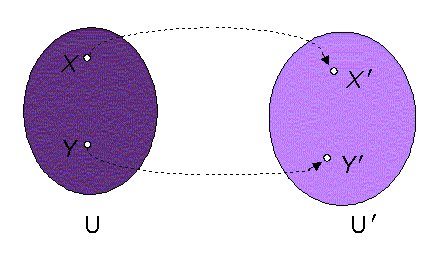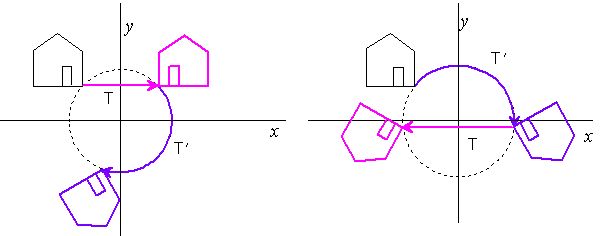# Geometric transformations

Transformation of space is a mapping of space to itself, in which any point in the space is attached the only one image - point in the same space, due to a certain determined rule.Figure 1: Mapping of sets

Regular transformation is a one-to-one mapping of the set U on the set U´, in which any element X of the set U (original $X\in \mathrm{U}$) is attached the only one element X ´ in the set U´ (image $X\text{'}\in \mathrm{U}\text{'}$) according to the rule:

1. $X\ne Y⇒X\text{'}\ne Y\text{'}$ , two different elements of set U are mapped to two different elements of set U',
2. $X\text{'}\ne Y\text{'}⇒X\ne Y$, two different elements of set U' are mapped to two different elements of set U.

If the sets U and U´ are geometric spaces with elements in points, regular transformations defined on the sets of points in these spaces are called geometric transformations. If U = U´, we speak about geometric transformations of space to itself.

Image of a geometric figure under the given geometric transformation is a set of images of all points of the given geometric figure. Geometric figures of space are mapped to geometric figures in the same space.

Properties of geometric figures that do not change under a specific geometric transformation are called invariant properties of the particular transformation.

All geometric transformations keep incidence as invariant property.

Geometric figures that are mapped to itself are called invariant under the respective transformation.

If all points of a geometric figure are invariant under a specific transformation, the figure is called point invariant under the respective transformation.

Geometric transformation is called linear, if the following property is satisfied:

1. image of any triple of non-coinciding points A , B , C located on one line is a triple of non-coinciding points A ´, B ´, C ´ also located on one line, image of a line in the space is a line.

Any linear transformation of the space can be defined by system of n equations determining the relations of the coordinates of two related points $X↦X\text{'}$, original $X=\left({x}_{1},{x}_{2},...,{x}_{n}\right)$ and its image $X\text{'}=\left({x}_{1}^{\text{'}},{x}_{2}^{\text{'}},...,{x}_{n}^{\text{'}}\right)$ in the form

 ${x}_{1}^{\text{'}}={a}_{11}{x}_{1}+{a}_{21}{x}_{2}+...+{a}_{n1}{x}_{n}$
 ${x}_{2}^{\text{'}}={a}_{12}{x}_{1}+{a}_{22}{x}_{2}+...+{a}_{n2}{x}_{n}$
 $....$
 ${x}_{1}^{\text{'}}={a}_{1n}{x}_{1}+{a}_{2n}{x}_{2}+...+{a}_{nn}{x}_{n}$

The system can be rewritten in the short form as

 $\left({x}_{1}^{\text{'}},{x}_{2}^{\text{'}},...,{x}_{n}^{\text{'}}\right)=\left({x}_{1},{x}_{2},...,{x}_{n}\right).\mathrm{T}$
 $X\text{'}=X.\mathrm{T}$

where T, matrix of the system, is the regular square matrix of rank n representing analytically the linear transformation

 $\mathrm{T}=\left(\begin{array}{cccc}{a}_{11}& {a}_{12}& ...& {a}_{1n}\\ {a}_{21}& {a}_{22}& ...& {a}_{2n}\\ .& .& .& .\\ {a}_{n1}& {a}_{n2}& ...& {a}_{nn}\end{array}\right)$

Determinant D of the matrix of linear geometric transformation is nonzero, $D=|\mathrm{T}|\ne 0$.

Geometric transformations can be composed forming thus a new composite transformation, while the composition of transformations is a non-commutative operation on the set of all linear transformations of the space. Composite transformation is represented by the matrix, which is the product of multiplication of matrices representing separate transformations.

Illustration of a composite transformation applied to a geometric figure is in the Figure 2. Symmetry above the axis y (trasnformation T) and revolution about the origin of the coordinate system by some angle (transformation T') are composed in two different ways, while the resulting image of the geometric figure under the two compositions differs, thus

 $\mathrm{T}.\mathrm{T}\text{'}\ne \mathrm{T}\text{'}.\mathrm{T}$Figure 2: Non-commutative composition of two transformations

Linear geometric transformations of space form a non-commutative group with well defined operation of composition, unit element of the group is identity I - transformation mapping all points of the space to itself, analytically represented by unit diagonal matrix

 $\mathrm{I}=\left(\begin{array}{cccc}1& 0& ...& 0\\ 0& 1& ...& 0\\ .& .& .& .\\ 0& 0& 0& 1\end{array}\right)$

Two transformations are called inverse transformations, if their composition is identity.

Classifying with respect to invariants of geometric transformations special groups of linear geometric transformations that are characteristic for separate geometric spaces can be defined.

Linear transformations of the space ${}_{\infty }{\mathrm{E}}^{3}\left(R\right)$, which keep invariant metric of the real subspace ${\mathrm{E}}^{3}\left(R\right)$ are called Euclidean metric transformations. They keep the ideal set of points at infinity as point invariant geometric figure.

Linear transformations of the space ${}_{\infty }{\mathrm{E}}^{3}\left(R\right)$, which keep the ideal set of points at infinity, but not as point invariant geometric figure, are called affine transformation. Parallelism is invariant property of affine transformations. Geometric figures keep parallelism invariant under affine transformations. Centre of a geometric figure is mapped to the centre of image of this geometric figure under an affine transformation.

Linear transformations of the space ${}_{\infty }{\mathrm{E}}^{3}\left(R\right)$, which keep invariant only incidence of geometric figures are called projective transformations. They can transform ideal points to real ones, and vice-versa, real points to infinity.